SSC BOARD PAPERS IMPORTANT TOPICS COVERED FOR BOARD EXAM 2024

### Practice Set 1.1, Linear Equation In Two Unknown Variables.

Practice Set 1.1

Complete the following activity to solve the simultaneous equations. 5x + 3y = 9 . (i)…

3a + 5b = 26; a + 5b = 22 Solve the following simultaneous equation.…

x + 7y = 10; 3x - 2y = 7 Solve the following simultaneous equation.…

2x - 3y = 9; 2x + y = 13 Solve the following simultaneous equation.…

5m - 3n = 19; m - 6n = -7 Solve the following simultaneous equation.…

5x + 2y = -3; x + 5y = 4 Solve the following simultaneous equation.…

1/3 x+y = 10/3 2x + 1/4 y = 11/4 Solve the following simultaneous equation.…

99x + 101 y = 499; 101x + 99y = 501 Solve the following simultaneous equation.…

49x - 57y = 172; 57x - 49y = 252 Solve the following simultaneous equation.…

###### Practice Set 1.1
Question 1.

Complete the following activity to solve the simultaneous equations.
5x + 3y = 9 ……. (i)
2x + 3y = 12 ……… (ii)

5x + 3y = 9 ……. (i)

2x + 3y = 12 ……… (ii)

Subtracting equation (ii) from (i), we get,

(5x + 3y ) - (2x + 3y) = 9 - 12

5x - 2x + 3y - 3y = -3

3x = -3

x = -1

Putting the value of x in equation (i),

5(-1) + 3y = 9

-5 + 3y = 9

3y = 14

y = 14/3

Let’s add equations (I) and (II).

Hence, x = -1 and y = 14/3 is the solution of the equation.

Question 2.

Solve the following simultaneous equation.

3a + 5b = 26; a + 5b = 22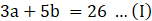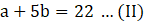Change the sign of Eq. (II)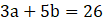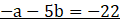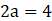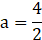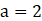Substituting a = 2 in Eq. (II)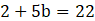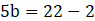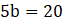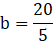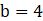∴ solution is (a, b) = (2, 4)

Question 3.

Solve the following simultaneous equation.

x + 7y = 10; 3x – 2y = 7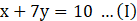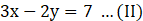Multiply Eq. I by 2 and Eq. II by 7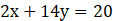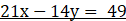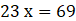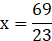x=3

Substituting x= 3 in Eq. I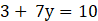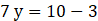7y=7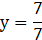y=1

∴ Solution is (x , y) = (3, 1)

Question 4.

Solve the following simultaneous equation.

2x – 3y = 9; 2x + y = 13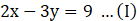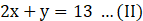Change the sign of Eq. (II)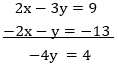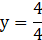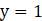Substituting y = 1 in Eq. (II)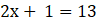2x = 13 - 1
2x = 12
x = 6

∴ solution is (x, y) = (1,6)

Question 5.

Solve the following simultaneous equation.

5m – 3n = 19; m – 6n = –7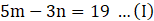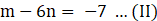Multiply Eq. II by 5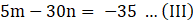equating (I) and (III), change the sign of Eq. (III)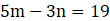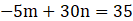⇒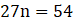⇒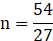⇒ n = 2

Substituting n = 2 in Eq 1
⇒ 5m - 3(2) = 19
⇒ 5m - 6 = 19
⇒ 5m = 25
⇒ m = 5

∴ Solution is (m , n) = (5, 2)

Question 6.

Solve the following simultaneous equation.

5x + 2y = –3; x + 5y = 4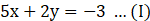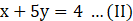Multiply Eq. I by 5 and Eq. II by 2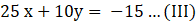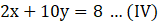Change sign of Eq.(IV)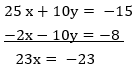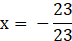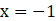Subsituting x=–1in Eq.II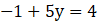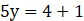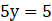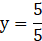∴ solution is (x, y) = (–1, 1)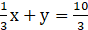⇒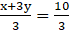⇒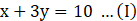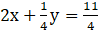⇒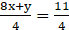⇒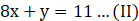Multiplying Eq. II by 3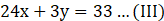Equating Eq. I and III, change the signs of Eq. III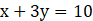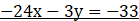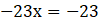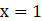Substituting x = 1 in Eq. I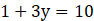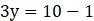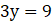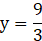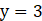∴ solution is (x,y) = (1, 3)

Question 8.

Solve the following simultaneous equation.

99x + 101 y = 499; 101x + 99y = 501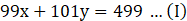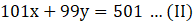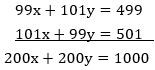Dividing both sides by 200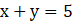…(III)

Subtract equation (I) and (II)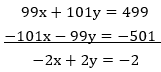Divide both sides by (–2)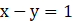…(IV)

Equating Eq. (III) and (IV)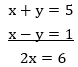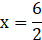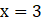Substituting x=3 in Eq. III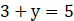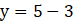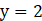∴ solution is (x, y) = (3, 2)

Question 9.

Solve the following simultaneous equation.

49x – 57y = 172; 57x – 49y = 252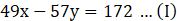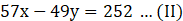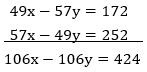Dividing both sides by 106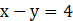…(III)

Subtract equation (I) and (II)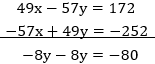Divide both sides by (–8)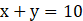…(IV)

Equating Eq. (III) and (IV)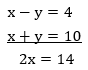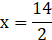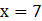Substituting x=7 in Eq. IV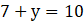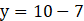∴ solution is (x, y) = (7,3)